## ML Aggarwal Class 10 Solutions for ICSE Maths Chapter 17 Mensuration Ex 17.1

These Solutions are part of ML Aggarwal Class 10 Solutions for ICSE Maths. Here we have given ML Aggarwal Class 10 Solutions for ICSE Maths Chapter 17 Mensuration Ex 17.1

More Exercise

Take π = $$\\ \frac { 22 }{ 7 }$$ unless stated otherwise.

Question 1.
Find the total surface area of a solid cylinder of radius 5 cm and height 10 cm. Leave your answer in terms of π.
Solution:
Radius of the cylinder (r) = 5 cm
Height (h) = 10 cm
Total surface area = 2πr (h + r)
= 2π x 5(10 + 5) cm2
= 10 x 15π
= 150π cm2

Question 2.
An electric geyser is cylindrical in shape, having a diameter of 35 cm and height 1.2m. Neglecting the thickness of its walls, calculate
(i) its outer lateral surface area,
(ii) its capacity in litres.
Solution:
Diameter of cylindrical geyser = 35 cm
Radius (r) = $$\\ \frac { 35 }{ 2 }$$ cm
Height = 1.2 m = 120 cm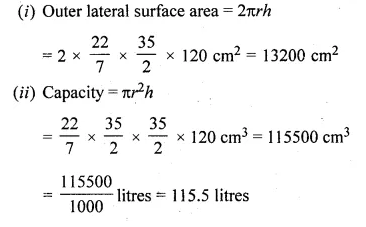Question 3.
A school provides milk to the students daily in cylindrical glasses of diameter 7 cm. If the glass is filled with milk upto a height of 12 cm, find how many litres of milk is needed to serve 1600 students.
Solution:
Number of students = 1600
Diameter of cylindrical glasses = 7 cm
Radius (r) = $$\\ \frac { 7 }{ 2 }$$ cm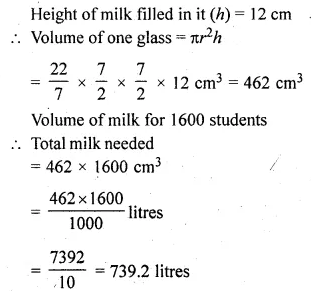Question 4.
In the given figure, a rectangular tin foil of size 22 cm by 16 cm is wrapped around to form a cylinder of height 16 cm. Find the volume of the cylinder.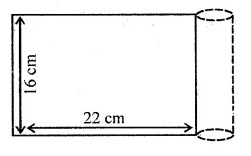Solution:
Length of rectangular tin foil (l) = 22 cm
and breadth (b) = 16 cm
By folding lengthwise, the radius of the cylinderQuestion 5.
(i) How many cubic metres of soil must be dug out to make a well 20 metres deep and 2 metres in diameter?
(ii) If the inner curved surface of the well in part (i) above is to be plastered at the rate of Rs 50 per m2, find the cost of plastering.
Solution:
(i) Depth of well (h) = 20 m
and diameter = 2 mQuestion 6.
A roadroller (in the shape of a cylinder) has a diameter 0.7 m and its width is 1.2 m. Find the least number of revolutions that the roller must make in order to level a playground of size 120 m by 44 m.
Solution:
Diameter of a road roller = 0.7 m
Radius (r) = $$\\ \frac { 0.7 }{ 2 }$$ = 0.35 m
and width (h) = 1.2 m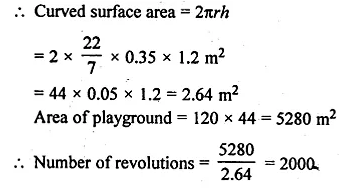Question 7.
If the volume of a cylinder of height 7 cm is 448 π cm3, find its lateral surface area and total surface area.
Solution:
Volume of a cylinder = 448 π cm3
Height (h) = 7 cm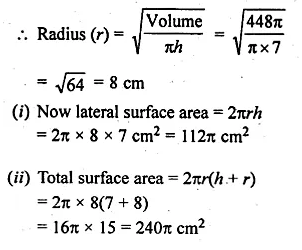Question 8.
A wooden pole is 7 m high and 20 cm in diameter. Find its weight if the wood weighs 225 kg per m3.
Solution:
Height of a wooden pole (h) = 7 m
Diameter = 20 cm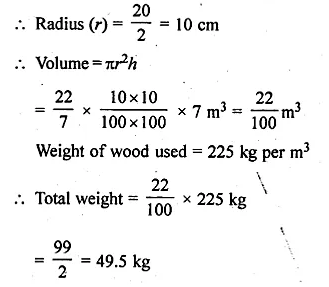Question 9.
The area of the curved surface of a cylinder is 4400 cm2, and the circumference of its base is 110 cm. Find
(i) the height of the cylinder.
(ii) the volume of the cylinder.
Solution:
Area of the curved surface of a cylinder = 4400 cm2
Circumference of base = 110 cm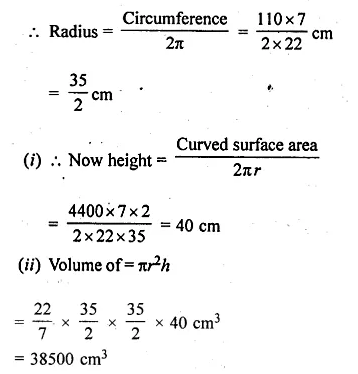Question 10.
A cylinder has a diameter of 20 cm. The area of curved surface is 1000 cm2. Find
(i) the height of the cylinder correct to one decimal place.
(ii) the volume of the cylinder correct to one decimal place. (Take π = 3.14)
Solution:
Diameter of a cylinder = 20 cm
Radius (r) = $$\\ \frac { 20 }{ 2 }$$ = 10 cm
Curved surface area = 1000 cm2Question 11.
The barrel of a fountain pen, cylindrical in shape, is 7 cm long and 5 mm in diameter. A full barrel of ink in the pen will be used up when writing 310 words on an average. How many words would use up a bottle of ink containing one-fifth of a litre?
Answer correct to the nearest. 100 words.
Solution:
Height of cylindrical barrel of a pen (h) = 7 cm
Diameter = 5 mm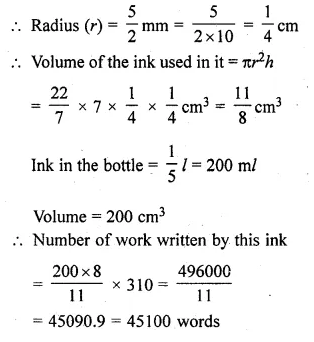Question 12.
Find the ratio between the total surface area of a cylinder to its curved surface area given that its height and radius are 7.5 cm and 3.5 cm.
Solution:
Radius of a cylinder (r) = 3.5 cm
and height (h) = 7.5 cm
Total surface area = 2πr(r + h)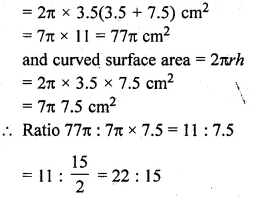Question 13.
The radius of the base of a right circular cylinder is halved and the height is doubled. What is the ratio of the volume of the new cylinder to that of the original cylinder?
Solution:
Let the radius of the base of a right circular cylinder = r
and height (h) = h
Volume = πr2h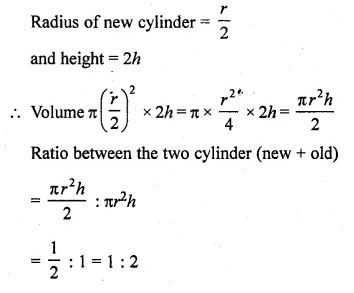Question 14.
(i) The sum of the radius and the height of a cylinder is 37 cm and the total surface area of the cylinder is 1628 cm2. Find the height and the volume of the cylinder.
(ii) The total surface area of a cylinder is 352 cm2. If its height is 10 cm, then find the diameter of the base.
Solution:
Sum of radius and height of a cylinder = 37 cm
Total surface area = 1628 cm2
Let r be radius and h be height, then r × h = 37
and 2πr(r + h) = 1628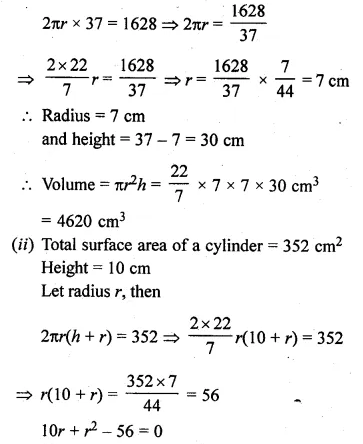Question 15.
The ratio between the curved surface and the total surface of a cylinder is 1 : 2. Find the volume of the cylinder, given that its total surface area is 616 cm2.
Solution:
Ratio between curved surface area and total surface area = 1 : 2
Total surface area = 616 cm2Question 16.
Two cylindrical jars contain the same amount of milk. If their diameters are in the ratio 3 : 4, find the ratio of their heights.
Solution:
Volume of two cylinders is the same
Diameter of both cylinder are in the ratio = 3 : 4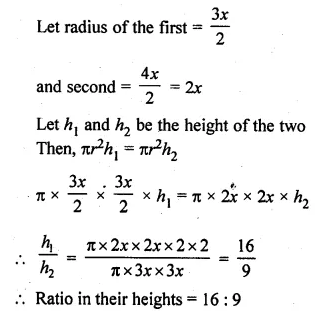Question 17.
A rectangular sheet of tin foil of size 30 cm x 18 cm can be rolled to form a cylinder in two ways along length and along breadth. Find the ratio of volumes of the two cylinders thus formed.
Solution:
Size of the sheet = 30 cm × 18 cm
(i) By rolling lengthwise,
The circumference of the cylinder = 2πr = 30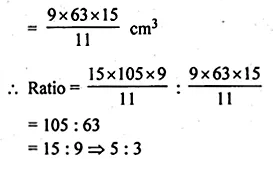Question 18.
A cylindrical tube open at both ends is made of metal. The internal diameter of the tube is 11.2 cm and its length is 21 cm. The metal thickness is 0.4 cm. Calculate the volume of the metal.
Solution:
Internal diameter of a metal tube = 11.2 cm
and radius (r) = $$\\ \frac { 11.2 }{ 2 }$$ = 5.6 cm
Length (h) = 21 cm
Thickness of metal = 0.4 cm
External radius (R) = 5.6 + 0.4 = 6.0 cmQuestion 19.
The given figure shows a metal pipe 77 cm long. The inner diameter of a cross-section is 4 cm and the outer one is 4.4 cm. Find its
(i) inner curved surface area
(ii) outer curved surface area
(iii) total surface area.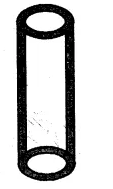Solution:
In the given figure,
Length of metal pipe (h) = 77 cm
Inner diameter = 4 cm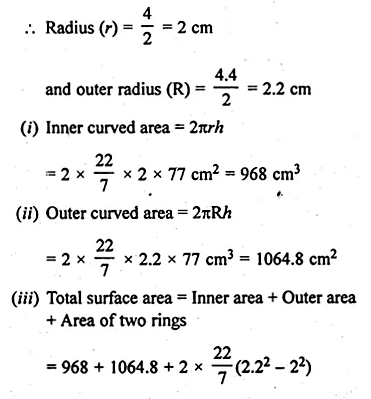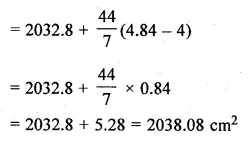Question 20.
A lead pencil consists of a cylinder of wood with a solid cylinder of graphite filled in the interior. The diameter of the pencil is 7 mm and the diameter of the graphite is 1 mm. If the length of the pencil is 14 cm, find the volume of the wood and that of the graphite.
Solution:
Diameter of the pencil = 7 mm
Radius (R) = $$\\ \frac { 7 }{ 2 }$$ mm = $$\\ \frac { 7 }{ 20 }$$ cm
Diameter of graphite (lead) = 1 mmQuestion 21.
A soft drink is available in two packs
(i) a tin can with a rectangular base of length 5 cm and width 4 cm, having a height of 15 cm and
(ii) a plastic cylinder with circular base of diameter 7 cm and height 10 cm. Which container has greater capacity and by how much?
Solution:
(i) Base of the tin of rectangular base = 5 cm × 4 cm
Height = 15 cm
Volume = lbh = 5 × 4 × 15 = 300 cm³
(ii) Base diameter of cylindrical plastic cylinder = 7 cmQuestion 22.
A cylindrical roller made of iron is 2 m long. Its inner diameter is 35 cm and the thickness is 7 cm all round. Find the weight of the roller in kg, if 1 cm³ of iron weighs 8 g.
Solution:
Length of cylindrical roller (h) = 2 m = 200 cm
Diameter = 35 cm
Inner radius = $$\\ \frac { 35 }{ 2 }$$ cm
Thickness = 7 cm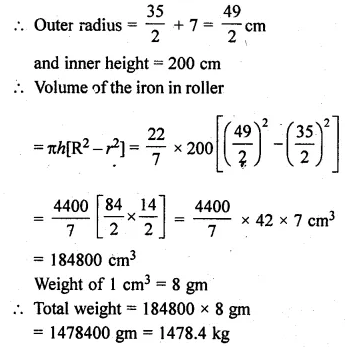We hope the ML Aggarwal Class 10 Solutions for ICSE Maths Chapter 17 Mensuration Ex 17.1 help you. If you have any query regarding ML Aggarwal Class 10 Solutions for ICSE Maths Chapter 17 Mensuration Ex 17.1, drop a comment below and we will get back to you at the earliest.